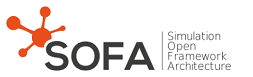SOFA API  98c952f5 Open source framework for multi-physics simuation
sofa::defaulttype::SolidTypes< R > Class Template Reference

`#include <SolidTypes.h>`

Inheritance diagram for sofa::defaulttype::SolidTypes< R >:[legend]

## Classes

class  ArticulatedInertia

class  RigidInertia

class  SpatialVector

class  Transform

class  Twist
A twist aka a SpatialVector representing a velocity This is pratically a SpatialVector (screw) with the additionnal semantics that this screw represents a twist (velocity) and not a wrench (force and torque) More...

class  Wrench
A wrench aka a SpatialVector representing a force and a torque This is pratically a SpatialVector (screw) with the additionnal semantics that this screw represents a wrench (force and torque) and not a twist (velocity) More...

## Static Public Member Functions

static Mat dyad (const Vec &u, const Vec &v)

static Vec mult (const Mat &m, const Vec &v)

static Vec multTrans (const Mat &m, const Vec &v)

static Mat crossM (const Vec &v)
Cross product matrix of a vector. More...

static ArticulatedInertia dyad (const SpatialVector &u, const SpatialVector &v)

static const char * Name ()

## Function details

template<class R = float>
 SolidTypes< R >::Mat3x3 sofa::defaulttype::SolidTypes< R >::crossM ( const Vec & v )
static

Cross product matrix of a vector.

template<class R >
 SolidTypes< R >::Mat3x3 sofa::defaulttype::SolidTypes< R >::dyad ( const Vec & u, const Vec & v )
static
template<class R >
 SolidTypes< R >::ArticulatedInertia sofa::defaulttype::SolidTypes< R >::dyad ( const SpatialVector & u, const SpatialVector & v )
static
template<class R = float>
 SolidTypes< R >::Vec sofa::defaulttype::SolidTypes< R >::mult ( const Mat & m, const Vec & v )
static
template<class R = float>
 SolidTypes< R >::Vec sofa::defaulttype::SolidTypes< R >::multTrans ( const Mat & m, const Vec & v )
static
template<class R = float>
 static const char* sofa::defaulttype::SolidTypes< R >::Name ( )
inlinestatic